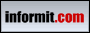Back to www.deitel.comISBN: 0-13-148398-6 © 2005 pages: 1576 Buy the Book!This tutorial introduces a new convenience feature of Java 5.0—the static import declaration—which enables programmers to refer to the imported static members of a class as if they were declared in the class that uses them. Thus, it is not necessary to qualify the imported static members with the name of the class in which they were declared. This tutorial is intended for students and professionals who are familiar with static class members in Java.

[Note: This tutorial is an excerpt (Section 8.12) of Chapter 8, Class and Objects: A Deeper Look, from our textbook Java How to Program, 6/e. This tutorial may refer to other chapters or sections of the book that are not included here. Permission Information: Deitel, Harvey M. and Paul J., JAVA HOW TO PROGRAM, ©2005, pp.380-382. Electronically reproduced by permission of Pearson Education, Inc., Upper Saddle River, New Jersey.]

# 8.12 static Import

In Section 6.3, you learned about the static fields and methods of class Math. We invoked class Math's static fields and methods by preceding each with the class name Math and a dot (.). A static import declaration (a new feature of J2SE 5.0) enables programmers to refer to imported static members as if they were declared in the class that uses them—the class name and a dot (.) are not required to use an imported static member.

A static import declaration has two forms—one that imports a particular static member (which is known as single static import) and one that imports all static members of a class (which is known as static import on demand). The following syntax imports a particular static member:

import static packageName.ClassName.staticMemberName;

where packageName is the package of the class (e.g., java.lang), ClassName is the name of the class (e.g., Math) and staticMemberName is the name of the static field or method (e.g., PI or abs). The following syntax imports all static members of a class:

import static packageName.ClassName.*;

where packageName is the package of the class (e.g., java.lang) and ClassName is the name of the class (e.g., Math). The asterisk (*) indicates that all static members of the specified class should be available for use in the class(es) declared in the file. Note that static import declarations import only static class members. Regular import statements should be used to specify the classes used in a program.

Figure 8.14 demonstrates a static import. Line 3 is a static import declaration, that imports all static fields and methods of class Math from package java.lang. Lines 9–12 access the Math class’s static field E (line 11) and the static methods sqrt (line 9), ceil (line 10), log (line 11) and cos (line 12) without preceding the field name or method names with class name Math and a dot.

 Fig. 8.14 Static import Math methods.
```   1  // Fig. 8.14: StaticImportTest.java   2  // Using static import to import static methods of class Math.   3  import static java.lang.Math.*;   4     5  public class StaticImportTest    6  {   7     public static void main( String args[] )    8     {   9        System.out.printf( "sqrt( 900.0 ) = %.1f\n", sqrt( 900.0 ) );  10        System.out.printf( "ceil( -9.8 ) = %.1f\n", ceil( -9.8 ) );  11        System.out.printf( "log( E ) = %.1f\n", log( E ) );  12        System.out.printf( "cos( 0.0 ) = %.1f\n", cos( 0.0 ) );  13     } // end main  14  } // end class StaticImportTest
```
 ` sqrt( 900.0 ) = 30.0ceil( -9.8 ) = -9.0log( E ) = 1.0cos( 0.0 ) = 1.0 `Common Programming Error 8.9 A compilation error occurs if a program attempts to import static methods that have the same signature or static fields that have the same name from two or more classes.

Return to Tutorial Index